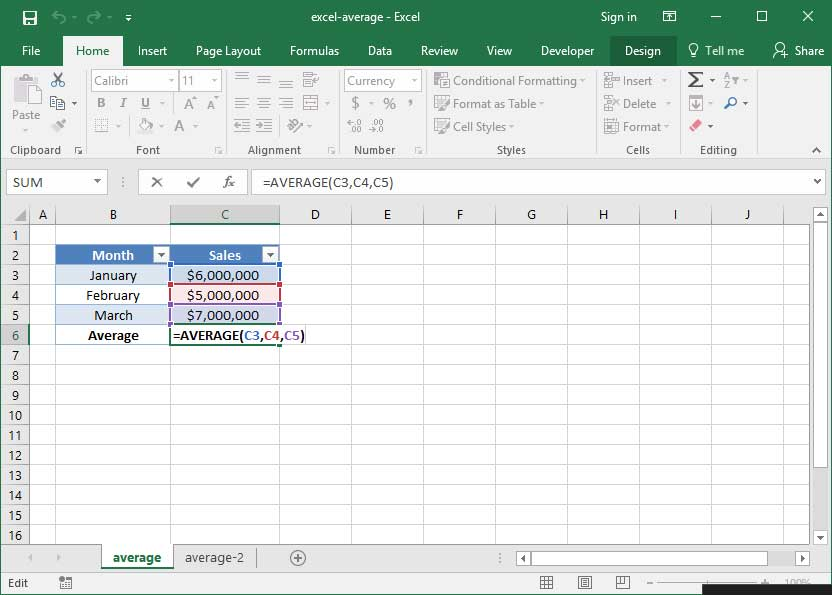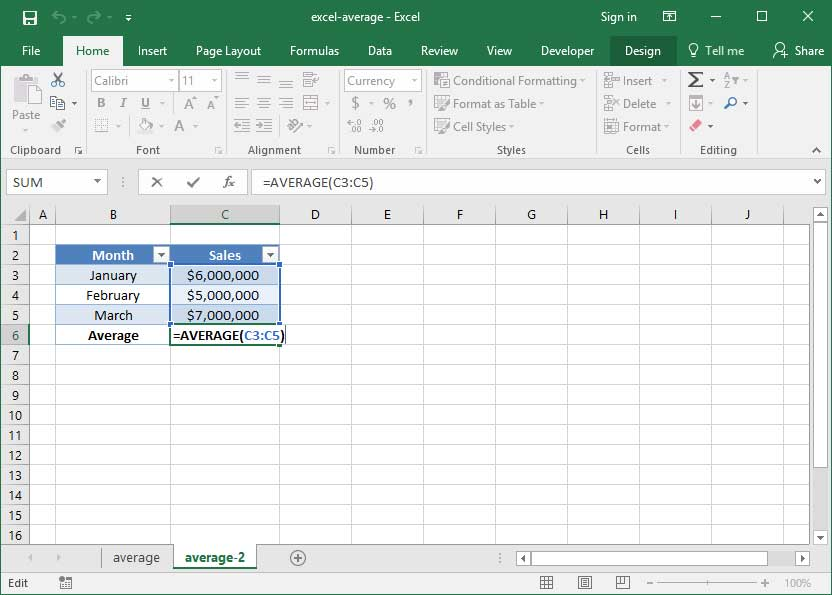# Using the AVERAGE formula in Excel

The next function we'll learn helps you calculate the average of a large set of numbers: AVERAGE. This is another commonly-used function in many worksheets. Like SUM, its mechanics are incredibly simple.

## Using AVERAGE

The basic syntax for the AVERAGE function is as follows:

`=AVERAGE(number_1, number_2...)`

Like SUM, the AVERAGE function can take as many arguments as you want to give it. Here are a couple examples of AVERAGE in action:

`=AVERAGE(3, 5)Output: 4`
`=AVERAGE(10, 20)Output: 15`
`=AVERAGE(0, 50,100, 150)Output: 75`

Like SUM, AVERAGE can be used with cell references rather than hard-coded numbers. Take, for example, the following sheet, in which we find SnackWorld's AVERAGE monthly sales for the months from January through March:`=AVERAGE(C3, C4, C5)Output: \$6,000,000`

The above formula outputs \$6,000,000, because the average of \$6,000,000, \$5,000,000, and \$7,000,000 is \$6,000,000.

## AVERAGE with a range of cells

The AVERAGE formula has one other key similarity to SUM: you can use it with a range of cells rather than individual cells to speed up formula writing when processing long lists. Let's take a look at how this works

In the below spreadsheet, we've rewritten our AVERAGE formula from the previous exercise to use a range of inputs rather than individual cells:`=AVERAGE(C3:C5)Output: \$6,000,000`

This formula also outputs \$6,000,000, because it performs the AVERAGE of all the cells in the range C3:C5.

## MEDIAN and MODE

Excel also contains simple functionality to calculate the mean and mode of a data range. Just replace your AVERAGE formula with a MEDIAN or MODE formula to use it!

## Errors with the AVERAGE function

If your AVERAGE function returns an error, it's likely because one of its inputs is an error like #VALUE! or #DIV/0!. If your function is returning one of these errors and you can't figure out why, try examining each of its inputs individually to ensure that they are all proper, real numbers.